# forecastTS

Forecast for a time series with trend, time varying seasonal, level shift and irregular component

## Syntax

• outFORE=forecastTS(outEST)example
• outFORE=forecastTS(outEST,Name,Value)example

## Description

forecastTS produces forecasts with confidence bands for a time series with trend (up to third order), seasonality (constant or of varying amplitude) with a different number of harmonics, level shift and explanatory variables.

 outFORE =forecastTS(outEST) Linear time varying seasonal component.

 outFORE =forecastTS(outEST, Name, Value) Quadratic trend and constant seasonal.

## Examples

expand all

###Linear time varying seasonal component.

close all
rng(1)
model=struct;
model.trend=1;
model.seasonal=103;
modelSIM=model;
modelSIM.trendb=[0 0];
modelSIM.seasonalb=40*[0.1 -0.5 0.2 -0.3 0.3 -0.1 0.222];
modelSIM.signal2noiseratio=20;
T=100;
% Simulate
outSIM=simulateTS(T,'model',modelSIM,'plots',1);
ySIM=outSIM.y;
% Estimate
outEST=LTSts(ySIM,'model',model,'plots',1);
% Forecast
outFORE=forecastTS(outEST,'model',model,'plots',1);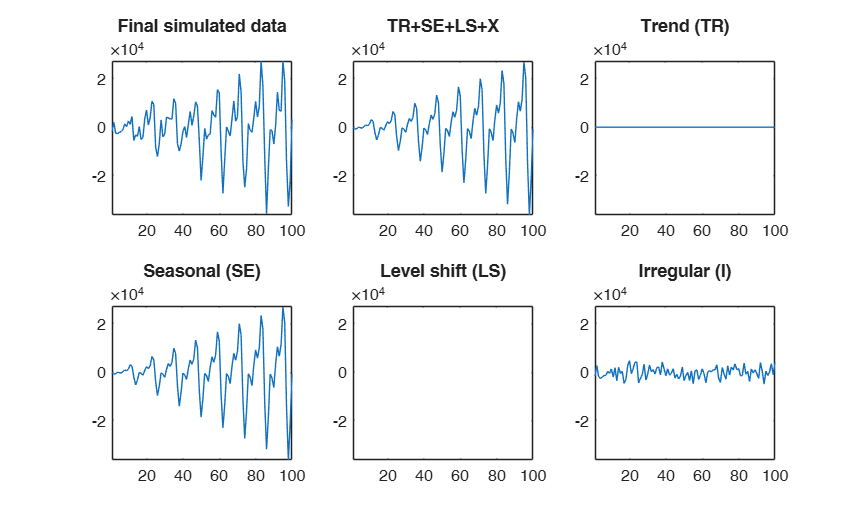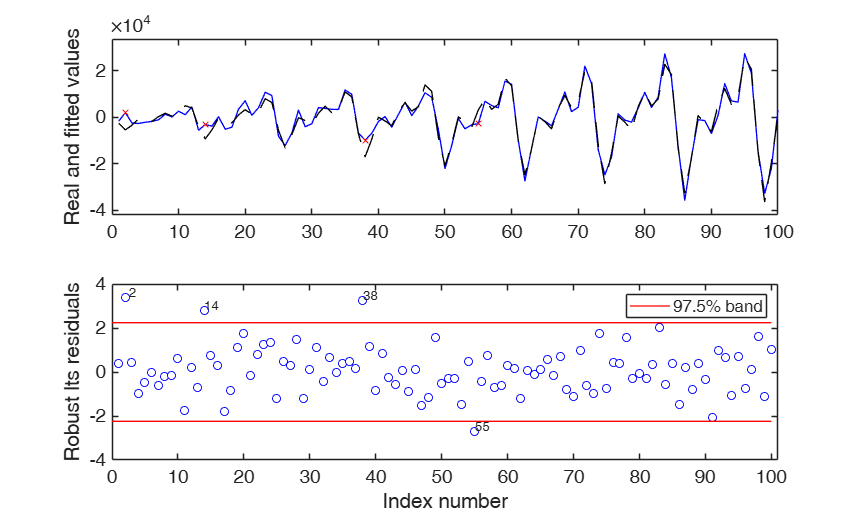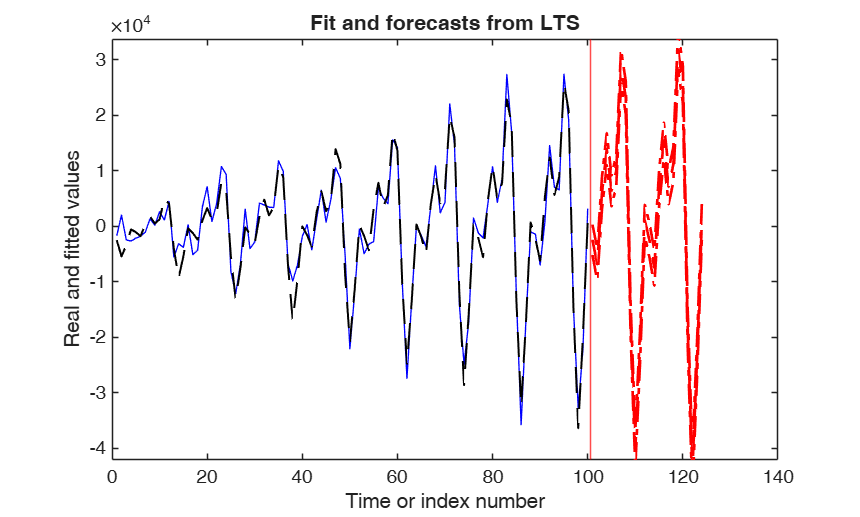###Quadratic trend and constant seasonal.

close all
rng(1)
model=struct;
model.trend=2;
model.seasonal=3;
modelSIM=model;
modelSIM.trendb=[100 10 -0.05];
modelSIM.seasonalb=400*[0.1 -0.5 0.2 -0.3 0.3 -0.1];
modelSIM.signal2noiseratio=1;
T=100;
% Simulate
outSIM=simulateTS(T,'model',modelSIM,'plots',1);
ySIM=outSIM.y;
% Estimate
outEST=LTSts(ySIM,'model',model,'plots',1);
% Forecast
outFORE=forecastTS(outEST,'model',model,'plots',1);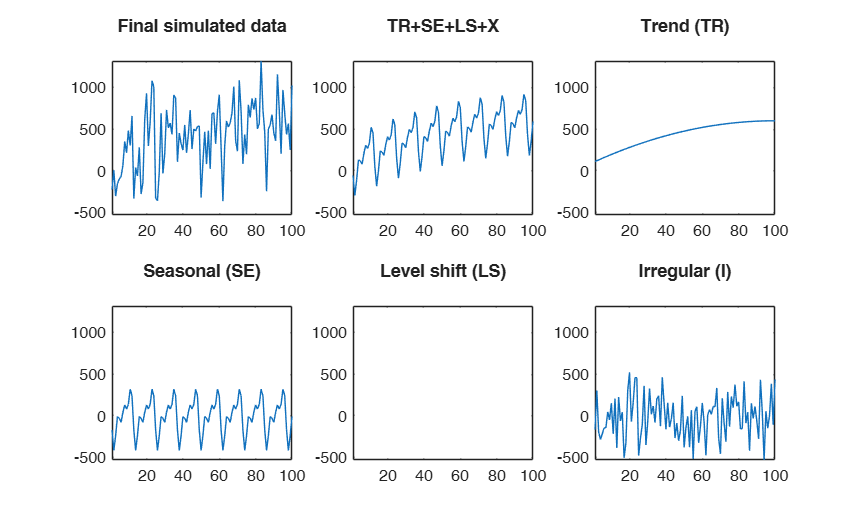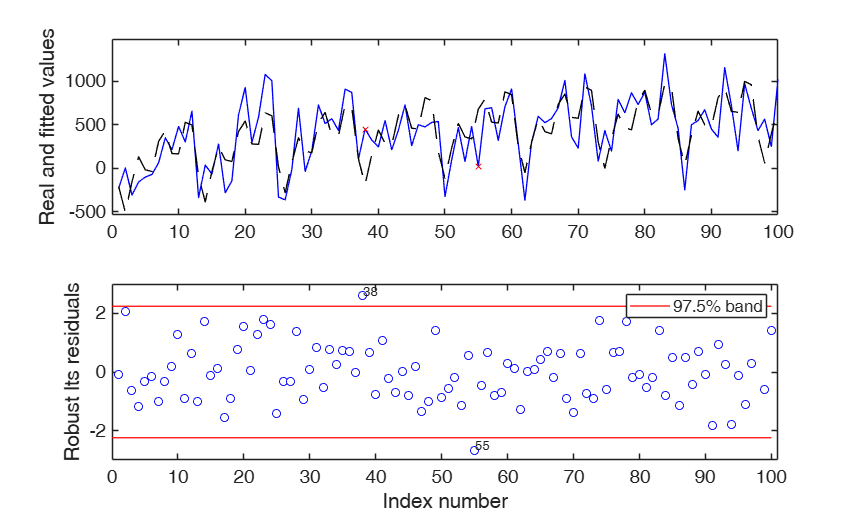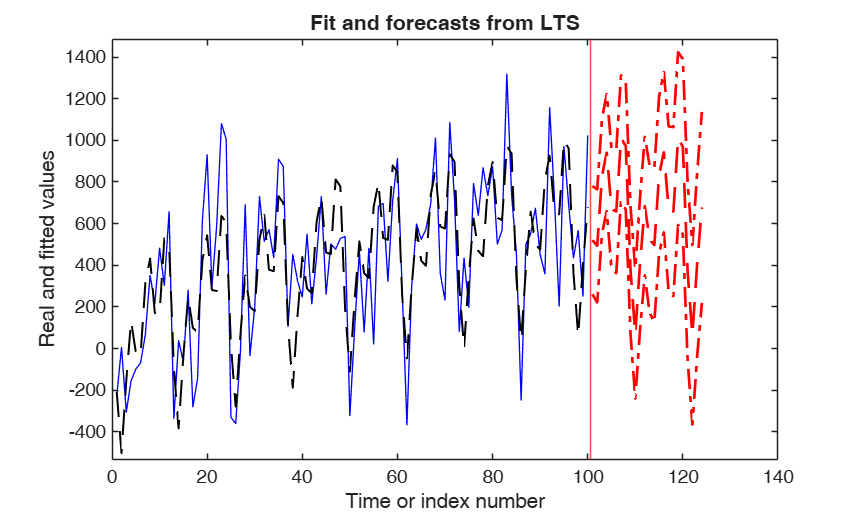## Related Examples

expand all

###Simulated time series with quadratic trend, fixed seasonal and level shift.

A time series of 100 observations is simulated from a model which contains a quadratic trend, a seasonal component with two harmonics no explanatory variables and a level shift in position 30 with size 5000 and a signal to noise ratio egual to 20

close all
rng(1)
model=struct;
model.trend=2;
model.seasonal=2;
model.lshift=30;
modelSIM=model;
modelSIM.trendb=[5,10,-3];
modelSIM.seasonalb=100*[2 4 0.1 8];
modelSIM.signal2noiseratio=20;
modelSIM.lshiftb=10000;
T=100;
% Simulate
outSIM=simulateTS(T,'model',modelSIM,'plots',1);
ySIM=outSIM.y;
% Estimate
%  model.lshift=5:T-5 implies that LS is investigated from position
% $5, 6, 7, ..., T-5$,
model.lshift=5:T-5;
outEST=LTSts(ySIM,'model',model,'plots',1,'msg',0);
% Forecast
outFORE=forecastTS(outEST,'model',model,'plots',1);###Contaminated airline data (1).

%   1949 1950 1951 1952 1953 1954 1955 1956 1957 1958 1959 1960
y = [112  115  145  171  196  204  242  284  315  340  360  417   % Jan
118  126  150  180  196  188  233  277  301  318  342  391    % Feb
132  141  178  193  236  235  267  317  356  362  406  419    % Mar
129  135  163  181  235  227  269  313  348  348  396  461    % Apr
121  125  172  183  229  234  270  318  355  363  420  472    % May
135  149  178  218  243  264  315  374  422  435  472  535    % Jun
148  170  199  230  264  302  364  413  465  491  548  622    % Jul
148  170  199  242  272  293  347  405  467  505  559  606    % Aug
136  158  184  209  237  259  312  355  404  404  463  508    % Sep
119  133  162  191  211  229  274  306  347  359  407  461    % Oct
104  114  146  172  180  203  237  271  305  310  362  390    % Nov
118  140  166  194  201  229  278  306  336  337  405  432 ]; % Dec
y=y(:);
% Contaminate the first 20 observations
y(1:20)=y(1:20)+200;
close all
% Model with linear trend, three harmonics for seasonal component and
% varying amplitude using a linear trend. Search for a level shift
model=struct;
model.trend=1;              % linear trend
model.s=12;                 % monthly time series
model.seasonal=103;         % three harmonics with linear time varying seasonality
model.lshift=10:140;            % search for level shift
out=LTSts(y,'model',model,'plots',1,'dispresults',true,'msg',0);
% 3 years forecasts
nfore=36;
StartDate=[1949 1];
conflev=0.999; % Wide confidence level for the forecast
outFORE=forecastTS(out,'model',model,'nfore',nfore,'StartDate',StartDate,'conflev',conflev);
                  Coeff        SE          t           pval
_________    _______    ________    ___________

b_trend1        301.9     3.3707      89.565    1.9432e-121
b_trend2       2.7697    0.03804      72.811    1.2536e-109
b_cos1       -0.94446      2.822    -0.33468        0.73839
b_sin1       -0.46559     1.3917    -0.33454         0.7385
b_cos2      -0.036471    0.11546    -0.31586         0.7526
b_sin2        0.55656     1.6636     0.33455        0.73849
b_cos3        0.22205    0.66425     0.33428        0.73869
b_sin3      -0.093486    0.28116     -0.3325        0.74003
b_varaml      0.58107     1.7666     0.32891        0.74273
b_lshift      -225.91     4.5415     -49.743     2.9417e-88

Level shift position t=21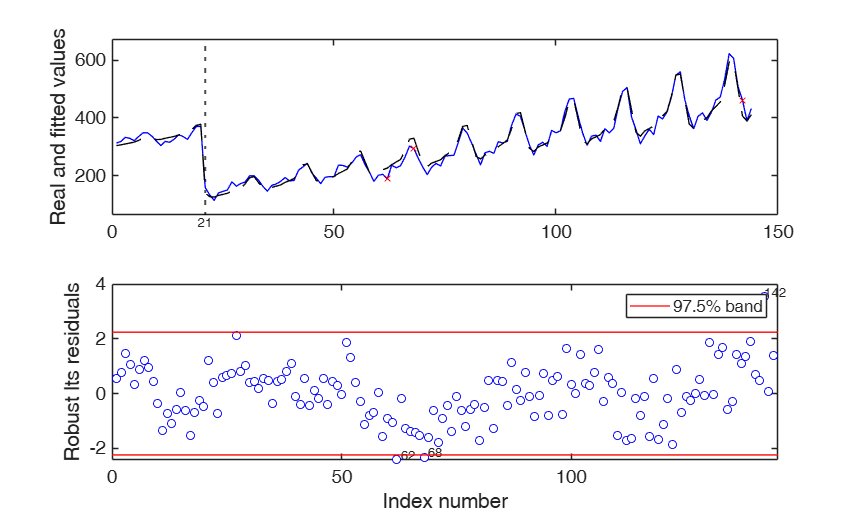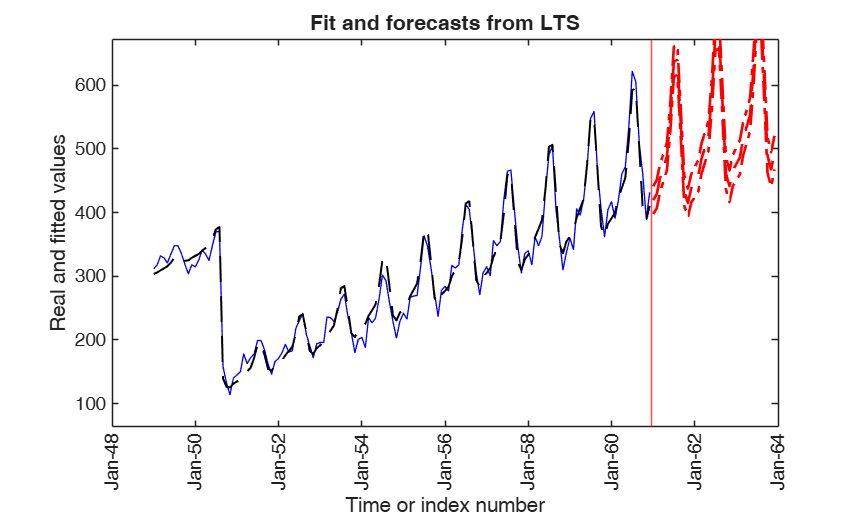###Contaminated airline data (2).

close all
% In this example we estimate a model without the seasonal component
%   1949 1950 1951 1952 1953 1954 1955 1956 1957 1958 1959 1960
y = [112  115  145  171  196  204  242  284  315  340  360  417   % Jan
118  126  150  180  196  188  233  277  301  318  342  391    % Feb
132  141  178  193  236  235  267  317  356  362  406  419    % Mar
129  135  163  181  235  227  269  313  348  348  396  461    % Apr
121  125  172  183  229  234  270  318  355  363  420  472    % May
135  149  178  218  243  264  315  374  422  435  472  535    % Jun
148  170  199  230  264  302  364  413  465  491  548  622    % Jul
148  170  199  242  272  293  347  405  467  505  559  606    % Aug
136  158  184  209  237  259  312  355  404  404  463  508    % Sep
119  133  162  191  211  229  274  306  347  359  407  461    % Oct
104  114  146  172  180  203  237  271  305  310  362  390    % Nov
118  140  166  194  201  229  278  306  336  337  405  432 ]; % Dec
y=y(:);
% Contaminate the first 20 observations
y(1:20)=y(1:20)+200;
% Model with linear trend and no seasonal component. Search for a level shift
model=struct;
model.trend=1;              % linear trend
model.s=12;                 % monthly time series
model.seasonal=0;          % no seasonal component
model.lshift=10:(length(y)-10);            % search for level shift
out=LTSts(y,'model',model,'plots',1,'dispresults',true,'msg',0);
% 3 years forecasts
nfore=36;
StartDate=[1949 1];
conflev=0.999; % Wide confidence level for the forecast
outFORE=forecastTS(out,'model',model,'nfore',nfore,'StartDate',StartDate,'conflev',conflev);
                 Coeff        SE          t          pval
_______    ________    _______    __________

b_trend1     307.43      7.2337       42.5    2.8696e-82
b_trend2     2.3919    0.087445     27.354    3.0083e-58
b_lshift    -214.71      9.7761    -21.963    2.3563e-47

Level shift position t=21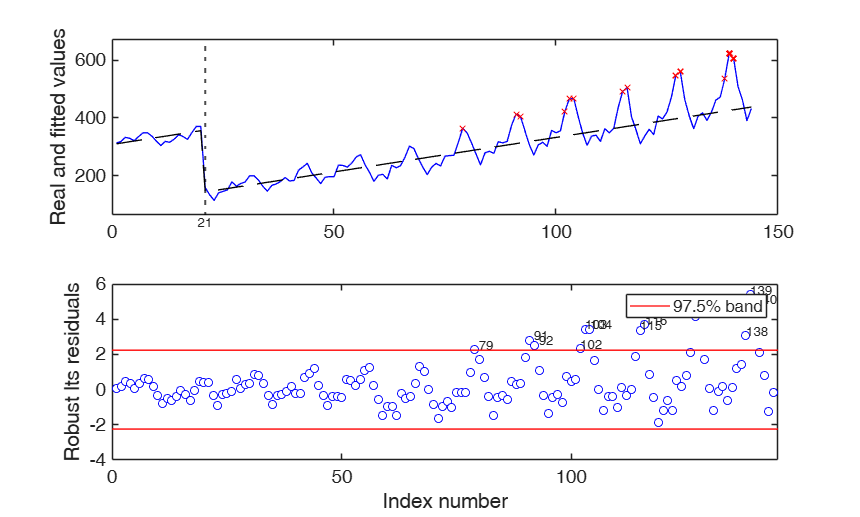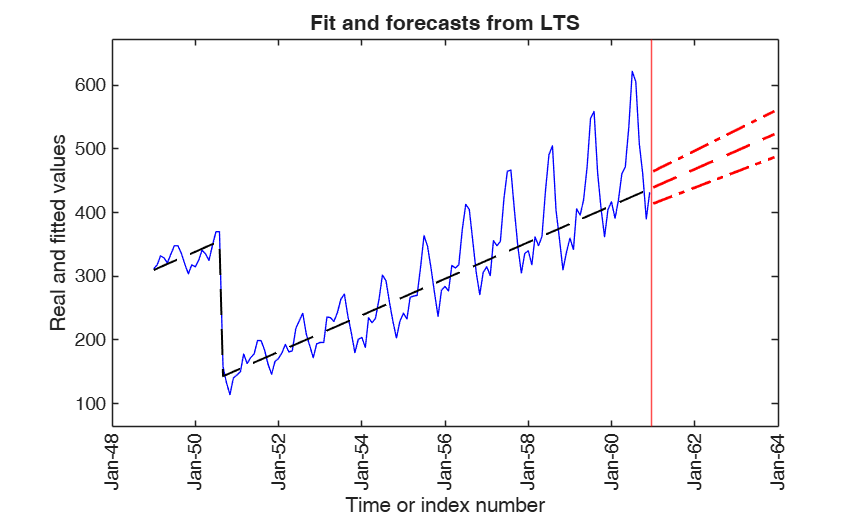### Example with simulated data.

Simulate data with linear trend, time varying seasonal component, and level shift

rng(1)
model=struct;
model.trend=1;
model.trendb=[1 1];
model.seasonal=103;
model.seasonalb=40*[0.5 -0.5 0.3 -0.3 0.1 -0.1 0.222];
model.lshift=40;
model.lshiftb=13000;
model.signal2noiseratio=20;
T=150;
FileNameOutput=[pwd filesep 'ysimout.txt'];
outSIM=simulateTS(T,'model',model,'FileNameOutput',FileNameOutput,...
'plots',true,'samescale',true);
y=outSIM.y;
% Data contamination
y(131:140)=y(131:140)-29000;
% Estimation
modelEST=struct;
modelEST.trend=1;
modelEST.seasonal=103;
modelEST.lshift=30:(length(y)-30);
outEST=LTSts(y,'model',modelEST,'dispresults',true,'plots',0);
% Forecasting
% nfore= number of forecasts;
nfore=36;
% Forecasts with a 99.9 per cent confidence level
OUTfore=forecastTS(outEST,'model',modelEST,'nfore',nfore,'conflev',0.999);

### Example of forecasting in a model with explanatory variables.

Simulated data with linear trend, varying seasonal and 1 explanatory variable.

rng(1000)
model=struct;
model.trend=1;
model.trendb=[5,1000];
model.seasonal=102;
model.seasonalb=100*[2 4 0.1 8 0.001];
model.signal2noiseratio=10;
T=120;
Xall=1e+2*randn(T,1);
model.X=Xall;
model.Xb=100;
out=simulateTS(T,'model',model,'plots',1);
% Fit a model using just the first 100 obs
yall=out.y;
Tred=100;
y=yall(1:Tred);
X=Xall(1:Tred,:);
model=struct;
model.trend=1;
model.seasonal=102;
% Potential level shift position is investigated in positions:
% t=11, t=11, ..., t=T-11.
model.lshift=-1;
model.X=X;
out=LTSts(y,'model',model,'plots',1,'dispresults',true);
% Note that in this case all the 120 values of Xall are supplied and
% the number of forecasts is 20
model.X=Xall;
forecastTS(out,'model',model,'nfore',20)

###Forecast with autoregressive components and expl var.

Simulated data with linear trend, varying seasonal and AR(2)

rng(1000)
model=struct;
model.trend=1;
model.trendb=[5,1000];
model.seasonal=102;
model.seasonalb=100*[2 4 0.1 8 0.001];
model.signal2noiseratio=10;
model.ARp=[1 2];
model.ARb=[0.2 0.7];
model.ARIMAX=true;
T=100;
out=simulateTS(T,'model',model,'plots',1);
% Fit a model imposing linear trend, sesonal component and AR(2)
y=out.y;
nfore=20;
Xall=1e+2*randn(T+nfore,1);
X=Xall(1:T,:);
model=struct;
model.trend=1;
model.seasonal=102;
% No level shift
model.lshift=0;
% Add a non important expl. variable
model.X=X;
model.ARp=[1 2];
out=LTSts(y,'model',model,'plots',1,'dispresults',true);
% Note that in this case all the 120 values of Xall are supplied and
% the number of forecasts is 20
model.X=Xall;
forecastTS(out,'model',model,'nfore',20)
Iterative search of sigmaeps depending on the desired signal2noise ratio.
After 1000 iterations, the value of signal2noise ratio closest to the desired one is 10.0005,
obtained with sigmaeps = 107955.6243
Coeff         SE           t           pval
_________    _________    ________    __________

b_trend1       -16801        33939    -0.49503       0.62178
b_trend2       2117.5       953.27      2.2214      0.028835
b_cos1         -32427        21648     -1.4979       0.13766
b_sin1          51362        27551      1.8642      0.065548
b_cos2         6181.2        16773     0.36852       0.71335
b_sin2         -39328        22569     -1.7426      0.084824
b_auto 1      0.16847     0.064852      2.5978       0.01096
b_auto 2      0.64325     0.065699      9.7907    7.7762e-16
b_explX1       360.92       157.24      2.2954      0.024035
b_varaml    0.0016604    0.0088853     0.18687       0.85218

ans =

struct with fields:

signal: [120×1 double]
trend: [120×1 double]
seasonal: [120×1 double]
X: [100×10 double]
lshift: 0
XAR: [120×1 double]
Xexpl: [120×0 double]
confband: [120×2 double]
datesnumeric: [120×1 double]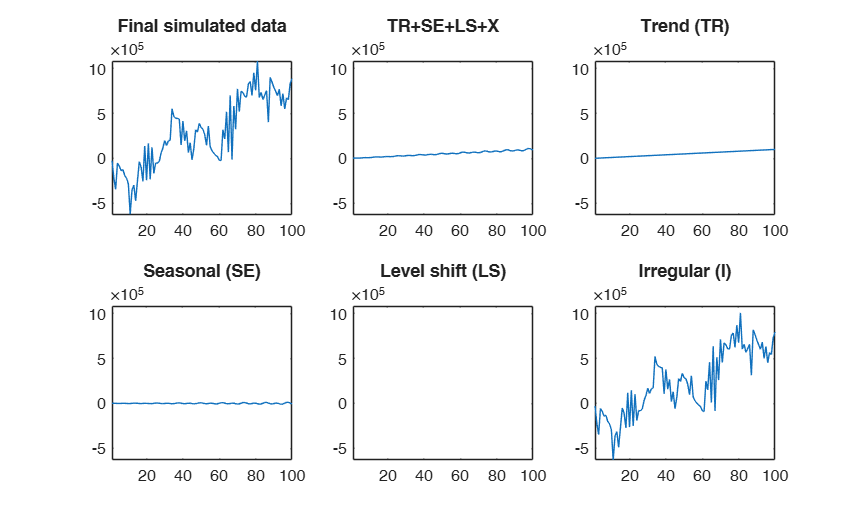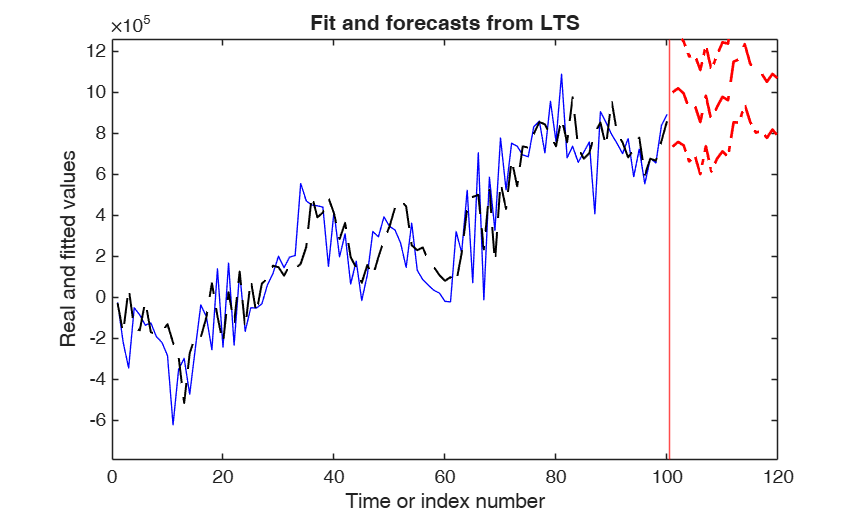### Check accuracy of forecasts.

AR(2) model with fixed seasonal

rng(1000)
model=struct;
model.trend=1;
model.trendb=[5,0.01];
model.seasonal=2;
model.seasonalb=0.1*[2 4 0.1 2];
model.signal2noiseratio=10;
model.ARp=[1 2];
model.ARb=[0.2 0.3];
model.ARIMAX=true;
T=150;
out=simulateTS(T,'model',model,'plots',1);
yall=out.y;
% Fit a model imposing linear trend, sesonal component and AR(2)
y=out.y(1:100);
nfore=50;
model=struct;
model.trend=1;
model.seasonal=2;
% No level shift
model.lshift=0;
model.ARp=[1 2];
out=LTSts(y,'model',model,'plots',1,'dispresults',true);
% Note that in this case all the 120 values of Xall are supplied and
% the number of forecasts is 20
forecastTS(out,'model',model,'nfore',50,'conflev',0.75)
hold('on')
plot(1:length(yall),yall)

## Input Arguments

### outEST — A structure containing the output of routine LTSts. Structure.

Structure containing the following fields.

Value Description
B

Matrix containing estimated beta coefficients, (including the intercept when options.intercept=true) standard errors, t-stat and p-values The content of matrix B is as follows: 1st col = beta coefficients The order of the beta coefficients is as follows: 1) trend elements (if present). If the trend is of order two there are r+1 coefficients if the intercept is present otherwise there are just r components;

2) linear part of seasonal component 2, 4, 6, ..., s-2, s-1 coefficients (if present);

3) coefficients associated with the matrix of explanatory variables and/or autoregressive part which have a potential effect on the time series under study (X);

4) non linear part of seasonal component, that is varying amplitude. If varying amplitude is of order k there are k coefficients (if present);

5) level shift component (if present). In this case there are two coefficients, the second (which is also the last element of vector beta) is an integer which specifies the time in which level shift takes place and the first (which is also the penultime element of vector beta) is a real number which identifies the magnitude of the upward (downward) level shift;

2nd col = standard errors;

3rd col = t-statistics;

4th col = p values.

posLS

scalar associated with best tentative level shift position. If this field does not exist, forecasts are done assuming no level shift.

invXX

$cov(\beta)/\hat \sigma^2$. p-by-p, square matrix.

If the model is linear out.invXX is equal to $(X'X)^{-1}$, else out.invXX is equal to $(A'A)^{-1}$ where $A$ is the matrix of partial derivatives. More precisely:

$a_{i,j}=\frac{\partial \eta_i(x_i, \hat \beta)}{\partial \hat \beta_j}$ where \begin{eqnarray} y_i & = & \eta(x_i,\beta)+ \epsilon_i \\ & = & \eta_i +\epsilon_i \\ & = & \eta(x_i,\hat \beta)+ e_i \\ & = & \hat \eta_i + e_i \end{eqnarray}

yhat

vector of n fitted values after final (NLS=non linear least squares) step: $(\hat \eta_1, \hat \eta_2, \ldots, \hat \eta_T)'$

scale

Final scale estimate of the residuals $\hat \sigma = cor \times \sum_{i \in S_m} [y_i- \eta(x_i,\hat \beta)]^2/(m-p)$

where $S_m$ is a set of cardinality $m$ which contains the units not declared as outliers and $p$ is the total number of estimated parameters and cor is a correction factor to make the estimator consistent.

REMARK: structure outEST can be conveniently created by function LTSts.

Data Types: struct

### Name-Value Pair Arguments

Specify optional comma-separated pairs of Name,Value arguments. Name is the argument name and Value is the corresponding value. Name must appear inside single quotes (' '). You can specify several name and value pair arguments in any order as  Name1,Value1,...,NameN,ValueN.

Example:  'model', model , 'nfore',12 , 'conflev',0.999 , 'plots',1 , 'titl','Plot with two wedges' , 'StartDate',[2016,3] , 'FileNameOutput',['C:' filesep 'myoutput' fielsep 'savesimdata.txt'] , 'dispresults',true 

### model —model type.structure.

A structure which specifies the model used to simulate the time series. The structure contains the following fields:

Value Description
trend

scalar (order of the trend component).

trend = 1 implies linear trend with intercept, trend = 2 implies quadratic trend, etc.

If this field is empty the simulated time series will not contain a trend. The default value of model.trend is 1.

s

scalar greater than zero which specifies the length of the seasonal period. For monthly data (default) s=12, for quartely data s=4, ...

The default value of model.s is 12 (that is monthly data are assumed)

seasonal

scalar (integer specifying number of frequencies, i.e. harmonics, in the seasonal component. Possible values for seasonal are $1, 2, ..., [s/2]$, where $[s/2]=floor(s/2)$.

For example: if seasonal = 1 (default) we have: $\beta_1 \cos( 2 \pi t/s) + \beta_2 sin ( 2 \pi t/s)$;

if seasonal = 2 we have: $\beta_1 \cos( 2 \pi t/s) + \beta_2 \sin ( 2 \pi t/s) + \beta_3 \cos(4 \pi t/s) + \beta_4 \sin (4 \pi t/s)$.

Note that when $s$ is even the sine term disappears for $j=s/2$ and so the maximum number of trigonometric parameters is $s-1$.

If seasonal is a number greater than 100 then it is possible to specify how the seasonal component grows over time.

For example, seasonal = 101 implies a seasonal component which just uses one frequency which grows linearly over time as follows: $(1+\beta_3 t)\times ( \beta_1 cos( 2 \pi t/s) + \beta_2 \sin ( 2 \pi t/s))$.

For example, seasonal = 201 implies a seasonal component which just uses one frequency which grows in a quadratic way over time as follows: $(1+\beta_3 t + \beta_4 t^2)\times( \beta_1 \cos( 2 \pi t/s) + \beta_2 \sin ( 2 \pi t/s))$.

If this field is an empty double (default) the simulated time series will not contain a seasonal component.

X

explanatory variabels. Matrix of size (length(y)+nfore)-by-nexpl.

If model.X is a matrix of size (length(y)+nfore)-by-nexpl, it contains the values of nexpl extra covariates which affect y for periods 1:(length(y)+nfore) where nfore is the requested number of forecasts. If this field is an empty double (default) there is no effect of explanatory variables.

ARp

vector with non negative integer numbers specifying the autoregressive components. For example: model.ARp=[1 2] means a AR(2) process;

model.ARp=2 means just the lag 2 component;

model.ARp=[1 2 5 8] means AR(2) + lag 5 + lag 8;

model.ARp=0 (default) means no autoregressive component.

ARtentout

matrix of size r-by-2 containing the list of the units declared as outliers (first column) and corresponding fitted values (second column) or empty scalar. If model.ARtentout is not empty, when the autoregressive component is present, the y values which are used to compute the autoregressive component are replaced by model.tentout(:,2) for the units contained in model.tentout(:,1)

Example:  'model', model 

Data Types: struct

### nfore —number of forecasts.scalar.

Positive integer which defines the number of forecasts. The default value of nfore is 24.

Example:  'nfore',12 

Data Types: double

### conflev —confidence level for the confidence bands.scalar.

A number between 0 and 1 which defines the confidence level which is used to produce the bands. The default value of conflev is 0.99.

Example:  'conflev',0.999 

Data Types: double

### plots —Plots on the screen.scalar.

If plots == 1 a plot with the real time series with fitted values and forecasts (with confidence bands) will appear on the screen. This plot is tagged forecastTS.

The default value of plot is 0, that is no plot is shown on the screen.

If plots == 2 an additional plot which shows the contribution of the different underlying components to fitted and forecasts will also appear on the screen. This plot is tagged decompTS. In other terms, in this plot we show the contribution given to outFORE.signal by outFORE.trend, outFORE.seasonal, outFORE.lshift, outFORE.Xexpl and outFORE.XAR. In all the underlying components (except trend) we impose the sum to zero constraint in the period 1:Tround where Tround=length(y)-mod(length(y),s);

Example:  'plots',1 

Data Types: double

### titl —Title.string.

A label for the title (default: 'Double wedge plot').

Example:  'titl','Plot with two wedges' 

Data Types: char

### StartDate —The time of the first observation.numeric vector of length 2.

Vector with two integers, which specify a natural time unit and a (1-based) number of samples into the time unit. For example, if model.s=12 (that is the data are monthly) and the first observation starts in March 2016, then StartDate=[2016,3]; Similarly, if models.s=4 (that is the data are quarterly) and the first observation starts in the second quarter or year 2014, then StartData=[2014,2]. The information in option StartDate will be used to create in the output the dates inside the time series object.

Example:  'StartDate',[2016,3] 

Data Types: double

### FileNameOutput —save simulated time series to txt file.character.

If FileNameOutput is empty (default) nothing is saved on the disk, else FileNameOutput will contain the path where to save the file on the disk.

Example:  'FileNameOutput',['C:' filesep 'myoutput' fielsep 'savesimdata.txt'] 

Data Types: Character

### dispresults —Display results of final fit.boolean.

If dispresults is true, labels of coefficients, estimated coefficients, standard errors, tstat and p-values are shown on the screen in a fully formatted way. The default value of dispresults is false.

Example:  'dispresults',true 

Data Types: logical

## Output Arguments

### outFORE — description Structure

Structure which contains the following fields:

Value Description
signal

vector of length (length(y)+nfore) containing predictive values in sample and out of sample.

Predictive values = TR+SE+XAR+Xexpl+LS.

Note that outFORE.signal =outFORE.trend+outFORE.seasonal +outFORE.lshift+outFORE.Xexpl+outFORE.XAR. The single components which make up the overall estimated values are given below.

trend

vector of length (length(y)+nfore) containing estimated trend (TR) in sample and out of sample.

If this component is not present, it is equal to 0.

seasonal

vector of length (length(y)+nfore) containing estimated seasonal component (SE) in sample and out of sample.

If this component is not present, it is equal to 0.

lshift

vector of length (length(y)+nfore) containing level shift (LS) in sample and out of sample.

If this component is not present, it is equal to 0.

Xexpl

vector of length (length(y)+nfore) containing the effect of the explanatory variables (Xexpl).

If this component is not present, it is equal to 0.

XAR

vector of length (length(y)+nfore) containing the effect of the explanatory variables (Xexpl).

If this component is not present, it is equal to 0.

confband

matrix of size (length(y)+nfore)-by-2 containing lower and upper confidence bands of the forecasts. The confidence level of the bands is specified in input parameter conflev. Note that the first length(y) rows of this matrix are equal to NaN.

datesnumeric

vector of length (length(y)+nfore) containing the dates in numeric format.

X

data matrix X containing trend, seasonal, expl and lshift, if the model is linear or linearized version of $\eta(x_i, \beta)$ if the model is non linear containing in the columns partial derivatives evaluated in correspondence of outEST.B(:,1) with respect to each parameter. In other words, the $i,j$-th element of outFORE.X is $\frac{\partial \eta_i(x_i, \hat \beta)}{\partial \hat \beta_j}$

$j=1, 2, \ldots, p$, $i=1, 2, \ldots, T$.

The size of this matrix is: T-by-p The field is present only if option yxsave is set to 1.

Rousseeuw, P.J., Perrotta D., Riani M. and Hubert, M. (2018), Robust Monitoring of Many Time Series with Application to Fraud Detection, "Econometrics and Statistics". [RPRH]